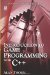# 1.5 Special Numbers

## 1.5 Special Numbers

The relationship between numbers has led to several special types being established in mathematics. These numbers have special properties. These are:

• Multiple-A multiple is a number that is some number of times greater than some other number. 10 is two times 5; 20 is two times 10. It is also worth noting that every number is a multiple of itself (5 × 1 = 5), and 0 is a multiple of every number (2 × 0 = 0).

• Parts and factors-A number can be said to be a part or parts of a larger number. 1 is one third part of 3. 2 is two third parts of 3. A number that is contained exactly inside another is called a factor. For example, 1, 2, 5, and 10 are said to be factors of 10 because each divides into 10 equally. 2 and 5 are also parts of 10. 2 is one fifth part of 10, and 5 is half of 10. Additionally, when two numbers are multiplied together to produce a product, say 7 × 2, the product is a multiple of both numbers and both numbers are factors of that product. So, 7 × 2 = 14 means 14 is a multiple of 7 and 2, and 7 and 2 are factors of 14. Furthermore, if 14 is then multiplied by some number, 7 and 2 will also be factors of that product. For example, 14 × 3 = 42. 42 is a multiple of 7, 2, and 14; therefore 7, 2, and 14 are certainly factors of 42.

• Even and odd numbers-If someone asks what the parity of a number is, the question is about whether a number is even or odd. Even numbers are those that are a multiple of 2 (like 2, 4, 6, 8, 10, ). The rest, which are not multiples of two, are called odd numbers (such as 3, 5, 7, 9, 11, ). If the last digit of a number is 0, 2, 4, 6, or 8 (such as 2,758), then it is even. If the last digit of a number is 1, 3, 5, 7, or 9, then it is odd.

• Prime numbers-A number is said to have primality when it is a prime number. There are an infinite number of prime numbers, and these numbers have only two factors: 1 and itself. In other words, if a number is chosen at random and, upon being divided by every number lower than itself, it can be shown that only 1 and itself divide into it equally, then it can be considered a prime number. The first few prime numbers are as follows: 2, 3, 5, 7, 11, and 13.Introduction to Game Programming with C++ (Wordware Game Developers Library)
ISBN: 1598220322
EAN: 2147483647
Year: 2007
Pages: 225
Authors: Alan Thorn

Similar book on Amazon

flylib.com © 2008-2017.
If you may any questions please contact us: flylib@qtcs.net